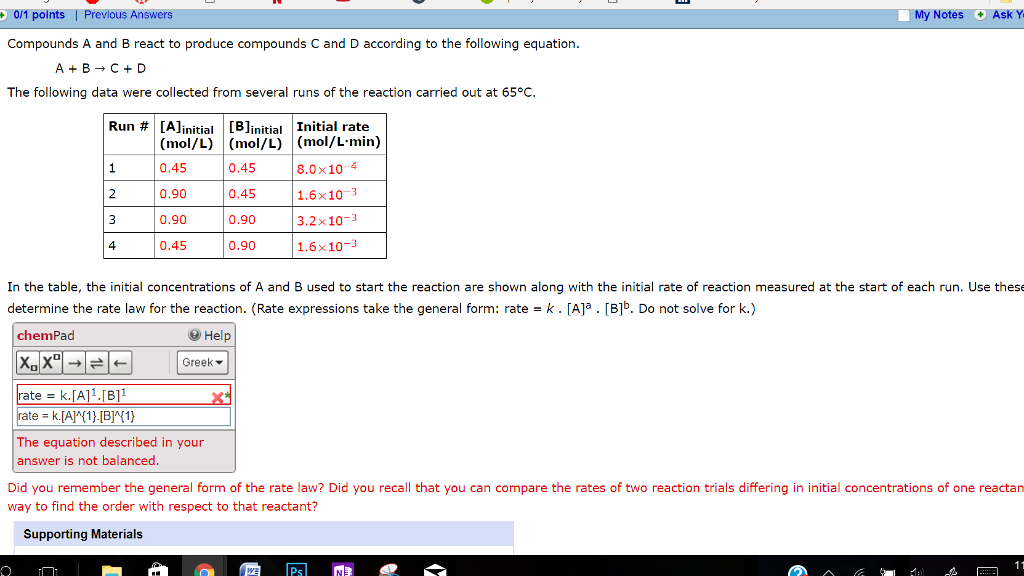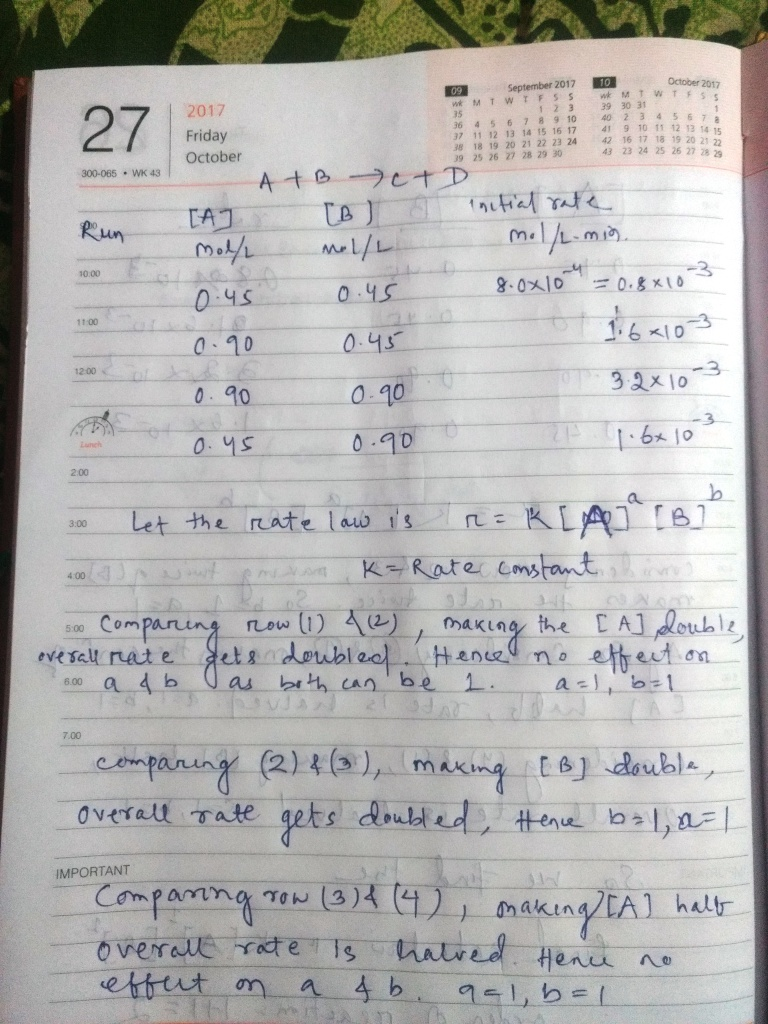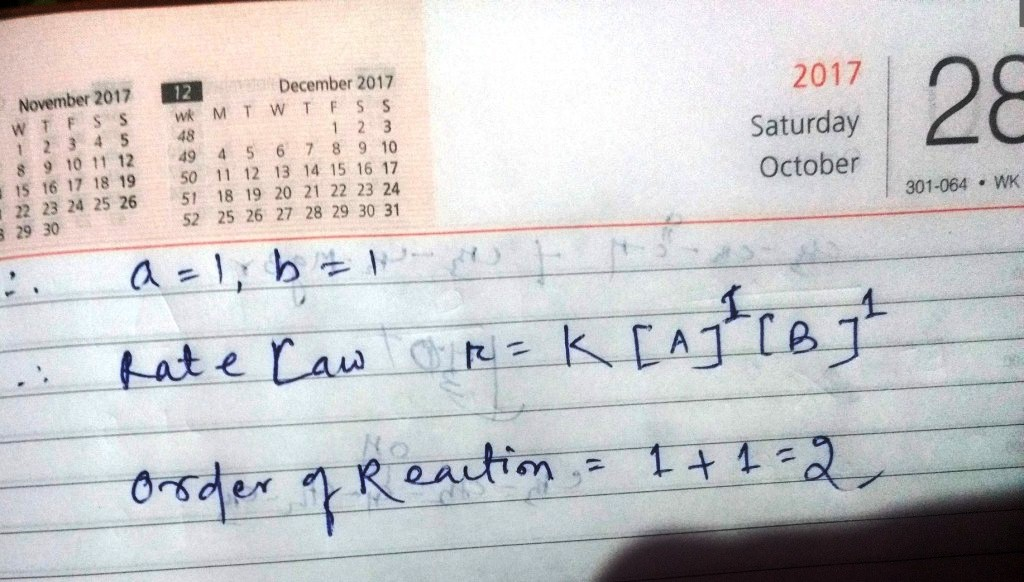# Question & Answer: Compounds A and B react to produce compounds C and D according to the following equation…..Compounds A and B react to produce compounds C and D according to the following equation. A + B rightarrow C + D The following data were collected from several runs of the reaction carried out at 65 degree C. In the table, the initial concentrations of A and B used to start the reaction are shown along with the initial rate of reaction measured at the start of each run. Use these determine the rate law for the reaction. (Rate expressions take the general form: rate = k middot [A]^a middot [B]^b. Do not solve for k.) Did you remember the general form of the rate law? Did you recall that you can compare the rates of two reaction trials differing in initial concentrations of one way to find the order with respect to that reactant?